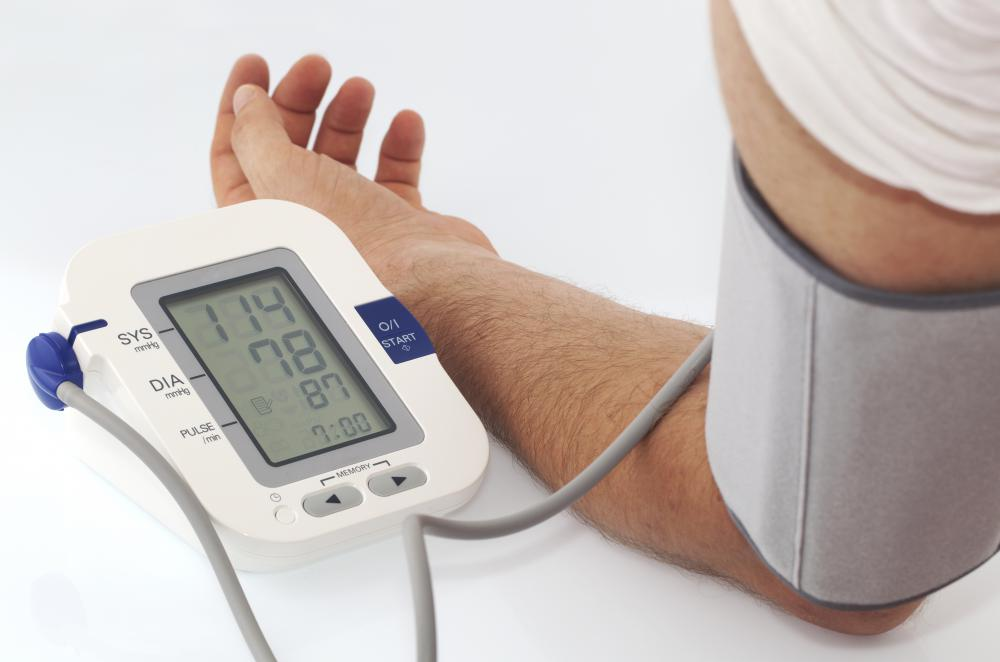# What is the Fatigue Index?

Elise Czajkowski

The fatigue index is a term most often used to describe a method for determining energy depletion during aerobic exercise. The index number indicates the rate at which an athlete's power output declines. It can be used as an indicator of an athlete's aerobic endurance.Stopwatches are used to measure sprint times when calculating the fatigue index.

A common way of determining an athlete's fatigue index is a sprinting test. The athlete will warm up for approximately ten minutes then be given a three to five minute break. She will then run six sprints of 30 to 35 meters, with a ten second break in between each sprint. The times for each of the sprints is recorded by a stopwatch.A sprinting test is a common way to determine an athlete's fatigue index.

To determine the athlete's fatigue index, the first thing to determine is the power output, in watts, of each sprint. This is determined by multiplying the velocity of the sprint by the force of the sprint. Velocity is determined by dividing the distance, in meters, by the time of the sprint.'Fatigue index' is used to describe a method for determining energy depletion during aerobic exercise.

To determine the force of the sprint, the acceleration, which is the velocity divided by the time of the sprint, must be determined. The acceleration of the sprint is multiplied by the weight of the athlete in kilograms. This number equals the force of the sprint. The fatigue index is found by subtracting the minimal power sprint from the maximal power sprint, then dividing by the total time of all six sprints.Distance runners need to have a much greater endurance than sprinters, who must use power to overcome the competition.

For example, an athlete who weighs 75 kilograms runs six sprints that are 30 meters each. Her times for the these sprints are as follows: 1 = 4.95 seconds, 2 = 5.10 seconds, 3 = 5.31 seconds, 4 = 5.59 seconds, 5 = 5.74 seconds, 6 = 5.98 seconds.

The power output of the first sprint is determined by the distance of the sprint, 30 meters, divided by the time taken to run it, which is 4.95 seconds. This gives the velocity: V = 30/4.95 = 6.06. To find the acceleration of the sprint, the velocity is divided by the time: A = 6.06/4.95 = 1.22. The force is equal to the athlete's weight multiplied by by the acceleration: F = 75 x 1.22 = 91.5. To find the power, the force is multiplied by the velocity: P = 91.5 x 6.06 = 554.49.

The power output from the athlete's first sprint is 554.49. As this was her fastest run, this will also be her maximal power output.

Using these equations, the power output for the second sprint is 507.15, the third sprint is 449.18, the fourth sprint is 386.64, the fifth sprint is 356.5, and the sixth sprint is 316.26. The sixth sprint is her minimal power output. To find the fatigue index, the total time of all the sprints together, which is 32.67 seconds, is needed.

The fatigue index is then found by subtracting 316.26 from 554.49 and dividing the result by 32.67: FI = (554.49 - 316.26)/32.67 = 7.29. Therefore, this athlete's fatigue index is 7.29.

An athlete with a high fatigue index, which is anything higher than 10, may need to work on her lactate tolerance. Lactate tolerance refers to a chemical compound the body produces during exercise. During strenuous power exercises, muscles produce more lactate than can be removed, so a high lactate tolerance allows for greater endurance.After completing cardiovascular exercises, an athlete typically experiences lower blood pressure than he had prior to starting the workout.

## You might also LikeAna1234

@Iluviaporos - Just remember that it is only one measurement for athletes. It uses sprinting deliberately, because sprinting burns glycogen and is something that no one can keep up indefinitely at the same level of power.

A person who relies on strength for their sport is probably not going to have the best results on the fatigue index, because they work on different things during training.

lluviaporos

@browncoat - I do wonder how many athletes would be considered very low on the fatigue index. I also kind of wonder whether it's possible for the average athlete to reach that level. It seems like the kind of thing that would be affected by training in high altitudes and other tricks like that.

browncoat

They made us do this in high school and called it the "beep test". It wasn't quite the same thing, but I know it was a way of measuring certain kinds of fitness and it must have done that by using the fatigue index or something similar.

It wasn't a set number of sprints, though. We were supposed to keep running at set intervals until we had to stop because of extreme fatigue. I usually dropped out pretty early, but I remember there were a couple of people who seemed like they could just keep going forever.